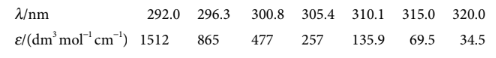×
Get Full Access to Atkins' Physical Chemistry - 11 Edition - Chapter 11a - Problem P11a.5
Get Full Access to Atkins' Physical Chemistry - 11 Edition - Chapter 11a - Problem P11a.5

×ISBN: 9780198769866 2042

## Solution for problem P11A.5 Chapter 11A

Atkins' Physical Chemistry | 11th Edition

• Textbook Solutions
• 2901 Step-by-step solutions solved by professors and subject experts
• Get 24/7 help from StudySoup virtual teaching assistantsAtkins' Physical Chemistry | 11th Edition

4 5 1 299 Reviews
28
5
Problem P11A.5

Ozone, uniquely among other abundant atmospheric constituents, absorbs ultraviolet radiation in a part of the electromagnetic spectrum energetic enough to disrupt DNA in biological organisms. This spectral range, which is denoted UV-B, spans from about 290nm to 320 nm. The molar extinction coefficient of ozone over this range is given in the table below (DeMore et al., Chemical kinetics and photochemical data for use in stratospheric modeling: Evaluation Number 11, JPL Publication 94–26 (1994)).Evaluate the integrated absorption coefficient of ozone over the wavelength range 290–320nm. Hint: $$\boldsymbol{\varepsilon}(\tilde{v})$$ can be fitted to an exponential function quite well.

Text Transcription:

varepsilontildev

Step-by-Step Solution:
Step 1 of 3

CHEM1200 Chapter 5 Parts 11-19 Professor: Mark Lord, Columbus State Community College The Gas Laws  Boyle’s Law-For a gas at constant TEMPRATURE and constant MASS, the volume of the gas will: o Increase as pressure decreases o Decrease as pressure increases  Charle’s Law- For a gas at constant PRESSURE and constant MASS, the volume of the gas will: o Increase as temperature increases o Decrease as temperature decreases  Gay-Lussac’s Law- For a gas at constant VOLUME and constant MASS, the temperature of the gas will: o Decrease as pressure decreases o Increase as pressure increases Combined Gas Law-Use when VOLUME, TEMPERATURE, or PRESSURE are changing (P1*V1)/T1=(P2*V2)/T2 P=PRESSURE (Any Unit) V=VOLUME (Any Unit) T=TEMPERRATURE (Kelvin ONLY) Avogadro’s Law-Relates volume of an ideal gas to changing mas at constant TEMPERATURE and PRESSURE  Gas at constant TEMPERATURE and PRESURE will increase in volume if the number of particles increases  Different gases at the same TEMPERATURE, PRESSURE, and VOLUME will contain the same amount of molecules and moles. PV=nRT P=PRESSURE (atm ONLY) V=VOLUME (Liters ONLY) T=TEMPERATURE (Kelvin ONLY) n=Amount (moles ONLY) R= 0.0821 L atm/ mol K (This number never changes) Dalton’s Law of Partial Pressures-Total pressure of a gas in a container (P-Total) is equal to the sum of the pressures of individual gases in gas mixtures.

Step 2 of 3

Step 3 of 3

## Discover and learn what students are asking

Calculus: Early Transcendental Functions : Introduction to Functions of Several Variables
?In Exercises 7-18, find and simplify the function values. $$f(x, y)=2 x+y^{2}$$ (a) $$\frac{f(x+\Delta x, y)-f(x, y)}{\Delta x}$$

Statistics: Informed Decisions Using Data : Discrete Random Variables
?What is a random variable?

Statistics: Informed Decisions Using Data : Comparing Three or More Means (One-Way Analysis of Variance)
?The following data represent a simple random sample of n = 4 from three populations that are known to be normally distributed. Verify that the F-test

Statistics: Informed Decisions Using Data : Testing the Significance of the Least-Squares Regression Model
?CEO Performance (Refer to Problem 31 in Section 4.1) The following data represent the total compensation for 12 randomly selected chief executive offi

Unlock Textbook Solution